Java帮帮-微信公众号Java帮帮-微信公众号将分享做到极致 支付宝小程序支付宝小程序支付也能阅读 程序员生活志-公众号程序员生活志-公众号程序员生活学习圈，互联网八卦黑料

 编程资讯
 Java
 程序员段子
 程序人生
 秒杀专栏
 名企资讯
 运维监控
 人事面试
 技术面试
 大禹编程
 UI
 SpringBoot
 SpringCloud(F版)
 SpringCloud(D版)
 spring
 Docker
 Dubbo
 IntelliJ IDEA
 Linux
 Nginx
 Tomcat
 Quartz
 微信小程序
 阿里云
 Python
 Java案例
 小程序源码
 Java源码
 手机资讯
 Sql Server
 MySQL
 MongoDB
 前端开发
 node.js
 CSS
 移动端
 jQuery
 Sublime Test
 JavaScript
 HTML
 Vue.js
 HTTPS
 分布式事物
 Redis
 JSON
 DevOps
 情感人生
 黑科技
 Oracle
 Git
 微服务
 大数据
 职业生涯
 人工智能
 Keras教程
 RocketMQ
 PyTorch教程
 Spring Security

# 第二天 变量&数据类型&运算符【悟空教程】

27702Java基础

# 第1章 变量

## 1.1 变量

### 1.1.1 变量概念### 1.1.3 变量的使用格式

• 变量类型   每一个变量均必须指定其变量类型。Java是强类型语言，在Java中不同的数据类型在内存中的存储方式是不尽相同的。所以必须在存储内容之前指定数据类型。

• 变量名   即使用标识符为变量起一个名字，之后在计算时，使用该名字即可参与计算。这个名字就代表了存储数值的空间。

• “=”号   这里的“=”号是运算符的一种，即=代表赋值运算，并非数学意义上的相等。

• 值   即真正变量存储的数值，需要与变量的数据类型一致。

/*

*/

public class Demo01Variable{

public static void main(String[] args){

//定义一个存储整数的变量

int myVar = 10;

//打印变量的值

System.out.println(myVar);//10

//改变变量的值

myVar = 20;

//打印变量的值

System.out.println(myVar);//20

}

}

### 1.1.4 日常变量使用的注意事项

• 可以同时定义多个变量

• 变量定义后可以不赋值，使用时再赋值。不赋值不能使用。

• 变量使用时有作用域的限制。

/*

*/

public class Demo02Variable{

public static void main(String[] args){

//可以同时定义多个变量

int a=5,b=10;

/*

int a = 5;

int b = 10;

*/

System.out.println(a);//5

System.out.println(b);//10

//变量定义后可以不赋值，使用时再赋值。不赋值不能使用。

int c;

//System.out.println(c);//错误: 可能尚未初始化变量c

//System.out.println(c=20);

c = 20;

System.out.println(c);

}

}

## 1.2 数据类型

Java是一种强类型语言，对不同数据结构进行了严格的区分，对每一种数据类型都做了明确定义，也同时规定了每一种数据类型的变量在内存中占用空间的大小。

### 1.2.1 字节

• 比特位

• 字节组成规则

• 常见的数据存储单位之间的换算

1B（字节） = 8bit

1KB = 1024B

1MB = 1024KB

1GB = 1024MB

1TB = 1024GB

### 1.2.2 数据类型分类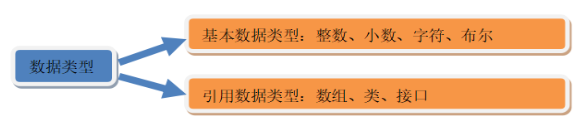• 整数型(精确)

int  占用4个字节  (整数的默认值)

byte  占用1个字节

short 占用2个字节

long  占用8个字节

long类型在赋值时，需要在值后加入字母l(L)区分其是一个长整型

• 浮点型(小数型，非精确，为近似值)

double 占用8个字节  (小数的默认值)

float  占用4个字节

float类型在赋值时，需要在值后加入字母f(F)区分其是一个浮点型

• 字符型

char  占用2个字节，必须使用’’包裹，且不能为空。

• 转义字符

\t  制表符

\’  单引号

\”  双引号

\\  反斜杠

• 字符存储原理

• 布尔型

boolean 占用1个字节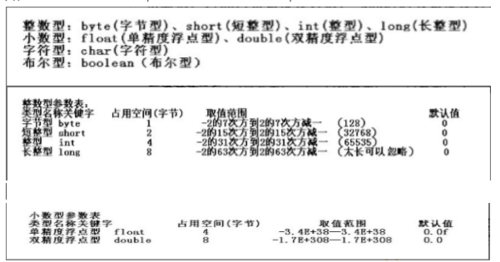类型 数据表示范围 整数类型byte -128～127 整数类型short -32768～32767 整数类型int(整数的默认值) -2147483648～2147483648 整数类型long -263～263-1 小数类型float -3.403E38～3.403E38 小数类型double(小数的默认值) -1.798E308～1.798E308 字符类型char 表示一个字符，如('a'，'A'，'0'，'家') 布尔类型boolean 只有两个值true与false

/*

byte:占用1个字节

short:占用2个字节

int:占用4个字节(默认)

long:占用8个字节,使用时必须在末尾加上一个小写字母l或者大写字母L

float:占用4个字节,使用时必须在末尾加上一个小写字母f或者大写字母F

double:占用8个字节(默认)

boolean:(true,false)占用1个字节

char:占用2个字节

*/

public class Demo03Variable{

public static void main(String[] args){

//整数

byte b = 100;

System.out.println(b);

short s = 10000;

System.out.println(s);

int i = 1600000000;

System.out.println(i);

long l = 6000000000L;

System.out.println(l);

//小数

double d = 3.14;

System.out.println(d);

float f = 2.6f;

System.out.println(f);

//小数是一个不精确的数

System.out.println(f-2);//0.5999999

//布尔

boolean b1 = true;

System.out.println(b1);

boolean b2 = false;

System.out.println(b2);

//字符

char c = 'a';

System.out.println(c);

//转义字符

char c1 = '\'';

System.out.println(c1);

char c2 = '\t';

System.out.println(c2+"Hello");

System.out.println('\\');

//字符实际存储的是一个数值

System.out.println(c+1);

}

}

### 1.2.3 数字类型转换

• 各种数据类型按照数据范围从小到大：

byte -> short -> int -> long -> float -> double

• 自动类型转换

double d = 1000;

int i = 100;

double d2 = i;

• 强制类型转换

int  i = (int)6.718;   //i的值为6

double  d = 3.14;

int  i2 = (int)d;     //i2的值为3

/*

byte < short(char)< int < long < float < double

*/

public class Demo04Variable{

public static void main(String[] args){

//自动类型转换 int --> double

int i = 100;

double d = i;

System.out.println(i);

System.out.println(d);

//强制类型转换

double d2 = 3.14;

//int i2 = d2;//错误: 可能损失精度

int i2 = (int)d2;

System.out.println(i2);

System.out.println(d2);

}

}

## 1.3 变量案例---超市水果报价单

### 1.3.1 案例介绍与演示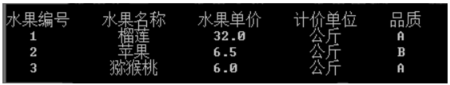### 1.3.2 案例分析

int liulianId  =  1;

String liulianName  =  “榴莲”;

double liulianPrice  =  32.0;

String liulianUnit  =  “公斤”;

char  liulianLevel = ‘A’;

int appleId =  2;

String appleName  =  “苹果”;

double applePrice  =  6.5;

String appleUnit  =  “公斤”;

char  appleLevel =  ‘B’;

System.out.println("水果编号    水果名称    水果单价    计价单位    品质");

System.out.println("   "+ liulianId  +"          "+ liulianName +"        "+

liulianPrice +"        "+ liulianUnit  +"       "+ liulianLevel);

System.out.println("   "+ appleId  +"          "+ appleName +"        "+

applePrice +"         "+ appleUnit  +"       "+ appleLevel);

/*

1   榴莲  32.0  公斤  A

2   苹果  6.5   公斤  B

3  猕猴桃  6.0   公斤  A

*/

public class List02{

public static void main(String[] args){

//定义榴莲的水果信息

int llId = 1;

String llName = "榴莲";

double llPrice = 32.0;

String llUnit = "公斤";

char llLevel = 'A';

//定义苹果的水果信息

int appleId = 2;

String appleName = "苹果";

double applePrice = 6.5;

String appleUnit = "公斤";

char appleLevel = 'B';

//定义猕猴桃的水果信息

int mhtId = 3;

String mhtName = "猕猴桃";

double mhtPrice = 6.0;

String mhtUnit = "公斤";

char mhtLevel = 'A';

System.out.println("水果编号水果名称水果单价计价单位品质");

System.out.println(llId+"  "+llName+"  "+llPrice+"  "+llUnit+"  "+llLevel);

System.out.println(appleId+"  "+appleName+"  "+applePrice+"  "+appleUnit+"  "+appleLevel);

System.out.println(3+" 猕猴桃  "+6.0+"  公斤  "+'A');

}

}

# 第2章 运算符

Int x = 3;

Int y = 4;

Int z = x + y

## 2.2 算数运算符

 运算符 运算规则 范例 结果 + 正号 +3 3 + 加 2+3 5 + 连接字符串 “中”+“国” “中国” - 负号 int a=3;-a -3 - 减 3-1 2 * 乘 2*3 6 / 除 5/2 2 % 取模(余数) 5%2 1 ++ 自增 int a=1;a++/++a 2 -- 自减 int b=3;a--/--a 2

• 加法运算符在连接字符串时要注意，只有直接与字符串相加才会转成字符串。

• 除法“/”当两边为整数时，取整数部分，舍余数。当两边为浮点型时，按正常规则相除。

• “%”为整除取余符号，小数取余没有意义。取余结果的符号与被取余数的符号相同。

• 得到的是两个相除数据的余数。

• 用处: 判断两个数据是否整除。

• 整数做被除数，0不能做除数，否则报错。

• 小数做被除数，整除0结果为Infinity，对0取模结果为NaN

• 自增与自减

1. 可以用于整数类型byte、short、int、long，浮点类型float、double，以及字符串类型char。

2. 在Java5.0及以上版本中，它们可以用于基本类型对应的包装器类Byte、Short、Integer、Long、Float、Double、Character。

3. 它们的运算结果的类型与被运算的变量的类型相同。

/*

+,-,*:相当于数学+,-*

+:做为字符串连接符

/:两个整数相除,结果是整数

%:两个整数取模(余数),结果是整数

++:自增(自身加1)

--:自减(自身减1)

1.加法运算符在连接字符串时要注意，只有直接与字符串相加才会转成字符串。

2.除法“/”当两边为整数时，取整数部分，舍余数。当两边为浮点型时，按正常规则相除。

3.%”为整除取余符号，小数取余没有意义。取余结果的符号与被取余数的符号相同。

4.整数做被除数，0不能做除数，否则报错。

5.小数做被除数，整除0结果为Infinity，对0取模结果为NaN

++- -为前置时，先+1-1，再使用值。

++- -为后置时，先使用值再+1-1

*/

public class Demo05Operator{

public static void main(String[] args){

//两个常量相加

System.out.println(3 + 5);//8

//变量加减乘除模

int a = 20;

int b = 7;

System.out.println(a + b);//27

System.out.println(a - b);//13

System.out.println(a * b);//140

System.out.println(a / b);//2

System.out.println(a % b);//6

//+:做为字符串连接符

System.out.println(""+"");//中国

/*

*/

//++:自增(自身加1)

//--:自减(自身减1)

int c = 10;

int d = 20;

//c++;

//d--;

++c;

--d;

System.out.println("c = " + c);//c = 11

System.out.println("d = " + d);//d = 19

System.out.println("===================================");

//1.加法运算符在连接字符串时要注意，只有直接与字符串相加才会转成字符串。

int e = 15;

int f = 20;

System.out.println("e + f = " + e + f);//e + f = 1520

System.out.println("e + f = " + (e + f));//e + f = 35

System.out.println(e + f + " = e + f");//35 = e + f

//2.除法“/”当两边为整数时，取整数部分，舍余数。当两边有浮点型时，按正常规则相除。

System.out.println(5.0 / 2);//2.5

//3.%”为整除取余符号，小数取余没有意义。取余结果的符号与被取余数(符号左边的数)的符号相同。

System.out.println(5.1 % 2);//1.0999999999999996

System.out.println(5 % 2);//1

System.out.println(-5 % 2);//-1

System.out.println(5 % -2);//1

System.out.println(-5 % -2);//-1

//4.整数做被除数，0不能做除数，否则报错。

//System.out.println(5 / 0);//ArithmeticException: / by zero

//5.小数做被除数，整除0结果为Infinity(无穷)，对0取模结果为NaN(not number 无效数字)

System.out.println(5.0 / 0);//Infinity

System.out.println(5.0 % 0);//NaN

System.out.println("===================================");

/*

++- -为前置时，先+1-1，再使用值。

++- -为后置时，先使用值再+1-1

*/

System.out.println(e);//15

System.out.println(f);//20

System.out.println(e++);//15

System.out.println(f--);//20

System.out.println(e);//16

System.out.println(f);//19

int i1 = 100;

int i2 = i1++ + 200;

System.out.println(i1);//101

System.out.println(i2);//300

int i3 = 100;

int i4 = ++i3 + 200;

System.out.println(i3);//101

System.out.println(i4);//301

}

}

## 2.3 赋值运算符

 运算符 运算规则 范例 结果 = 赋值 int a=2 2 += 加后赋值 int a=2，a+=2 4 -= 减后赋值 int a=2，a-=2 0 *= 乘后赋值 int a=2，a*=2 4 /= 整除后赋值 int a=2，a/=2 1 %= 取模后赋值 int a=2，a%=2 0

• 诸如+=这样形式的赋值运算符，会将结果自动强转成等号左边的数据类型。

/*

=,+=,-=,*=,/=,%=

+=,-=,*=,/=,%=运算符的运算规则:

+=,-=,*=,/=,%=注意事项:运算符左边必须是一个变量

+=,-=,*=,/=,%=特点:隐含一个强制类型转换

*/

public class Demo06Operator{

public static void main(String[] args){

int a = 10;

a += 20;//相当于 a = a + 20;

System.out.println("a = " + a);

//5 += 6;//错误: 意外的类型,这里5的位置需要一个变量

byte b = 10;

//b = b + 100;// 错误: 可能损失精度

//b = (byte)(b + 100);

b += 100;//+=隐含了一个强制类型转换 相当于b = (byte)(b + 100);

System.out.println("b = " + b);

}

}

## 2.4 比较运算符

• 又叫关系运算符，即判断两个操作数的大小关系及是否相等关系，比较运算符的返回一定为布尔值。

 运算符 运算规则 范例 结果 == 相等于 4==3 false != 不等于 4!=3 true < 小于 4<3 false > 大于 4>3 true <= 小于等于 4<=3 false >= 大于等于 4>=3 true
• ==，!=，>，<，>=，<=

• 关系运算符的结果都是boolean型，也就是要么是true，要么是false。

• 注意事项

• n 关系运算符“==”不能误写成“=” 。

public class Demo06Operator {

public static void main(String[] args) {

int a = 10;

int b = 20;

int c = 10;

System.out.println(a == b);

System.out.println(a == c);

System.out.println("-----------------");

System.out.println(a != b);

System.out.println(a != c);

System.out.println("-----------------");

System.out.println(a > b);

System.out.println(a > c);

System.out.println("-----------------");

System.out.println(a >= b);

System.out.println(a >= c);

System.out.println("-----------------");

int x = 3;

int y = 4;

// System.out.println(x == y);

// System.out.println(x = y);// y赋值给x，把x的值输出

boolean bb = (x == y);

// 报错

// boolean cc = (x = y);

int cc = (x = y);

}

}

## 2.5 逻辑运算符

 运算符 运算规则 范例 结果 & 与(并且) false&true False | 或 false|true True ^ 异或 true^flase True ! 非(取反) !true Flase && 短路与 false&&true False || 短路或 true ||true True
• 作用:逻辑运算符用于连接布尔型表达式，在Java中不可以写成3<x<6，应该写成x>3 && x<6 。

• 基本使用: &(与),|(或),^(异或),!(非)

• 与：& 有false则false

• 或：| 有true则true

• 异或：^ 相同则false,不同则true

• 非：! true则false,false则true

public class Demo08Operator {

public static void main(String[] args) {

int a = 10;

int b = 20;

int c = 30;

System.out.println((a > b) & (a > c));// false & false

System.out.println((a < b) & (a > c)); // true & false

System.out.println((a > b) & (a < c)); // false & true

System.out.println((a < b) & (a < c)); // true & true

System.out.println("---------------");

System.out.println((a > b) | (a > c));// false | false

System.out.println((a < b) | (a > c)); // true | false

System.out.println((a > b) | (a < c)); // false | true

System.out.println((a < b) | (a < c)); // true | true

System.out.println("---------------");

System.out.println((a > b) ^ (a > c));// false ^ false

System.out.println((a < b) ^ (a > c)); // true ^ false

System.out.println((a > b) ^ (a < c)); // false ^ true

System.out.println((a < b) ^ (a < c)); // true ^ true

System.out.println("---------------");

System.out.println((a > b)); // false

System.out.println(!(a > b)); // !false

System.out.println(!!(a > b)); // !!false

}

}

• &&和&的区别

• 单&时，左边无论真假，右边都进行运算；

• 双&时，如果左边为真，右边参与运算，如果左边为假，那么右边不参与运算。

• “|”和“||”的区别同理，双或时，左边为真，右边不参与运算。

public class Demo09Operator {

public static void main(String[] args) {

int a = 10;

int b = 20;

int c = 30;

System.out.println((a > b) && (a > c));// false && false

System.out.println((a < b) && (a > c)); // true && false

System.out.println((a > b) && (a < c)); // false && true

System.out.println((a < b) && (a < c)); // true && true

System.out.println("---------------");

System.out.println((a > b) || (a > c));// false || false

System.out.println((a < b) || (a > c)); // true || false

System.out.println((a > b) || (a < c)); // false || true

System.out.println((a < b) || (a < c)); // true || true

System.out.println("---------------");

int x = 3;

int y = 4;

// System.out.println((x++ > 4) & (y++ > 5)); // false & false

System.out.println((x++ > 4) && (y++ > 5)); // false && false

System.out.println("x:" + x);

System.out.println("y:" + y);

}

}

## 2.6 三元运算符

• (条件表达式)？表达式1：表达式2；

• 表达式：通俗的说，即通过使用运算符将操作数联系起来的式子，例如：

• 3+2，使用算数运算符将操作数联系起来，这种情况，我们称为算数表达式。

• 3>2，使用比较运算符（也称为条件运算符）将操作数联系起来，这种情况，我们称为条件表达式。

• 其他表达式，不再一一举例。

• 三元运算符运算规则：

• 三元运算符，最终一定会产生一个结果值，这个值必须被使用起来。

• 要么被运算符使用，要么被打印

/*

(条件表达式) ? 表达式1 : 表达式2;

true:表达式1作为3元运算符的结果

false:表达式2作为3元运算符的结果

*/

public class Demo10Operator{

public static void main(String[] args){

int a = 10;

int b = 20;

int max = (a > b) ? 10 : 20;

System.out.println("max = " + max);

int c = 15;

int d = 10;

//System.out.println((c != d) ? "相等" : "不相等");

String s = (c != d) ? "相等" : "不相等";

System.out.println("s = " + s);

//(c != d) ? "相等" : "不相等";//错误: 不是语句

//3;//错误: 不是语句

}

}

## 2.7 运算符优先级

### 2.7.2 优先级规则

 优先级 描述 运算符 1 括号 ()、[] 2 正负号 +、- 3 自增自减，非 ++、--、! 4 乘除，取余 *、/、% 5 加减 +、- 6 移位运算 <<、>>、>>> 7 大小关系 >、>=、<、<= 8 相等关系 ==、!= 9 按位与 & 10 按位异或 ^ 11 按位或 | 12 逻辑与 && 13 逻辑或 || 14 条件运算 ?: 15 赋值运算 =、+=、-=、*=、/=、%= 16 位赋值运算 &=、|=、<<=、>>=、>>>=

## 2.8 运算符案例---超市购物小票案例

### 2.8.1 案例介绍与演示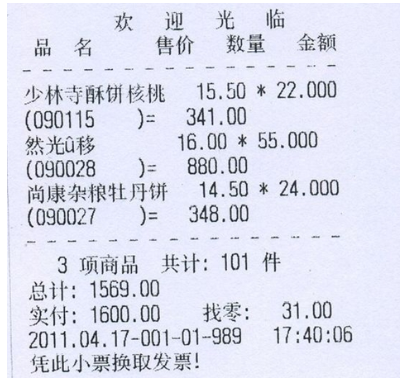### 2.8.2 案例分析

a) 总体思路

• 小票界面结构分析：通过小票界面分析，可以将复杂的问题简单化，规划出来更为具体的需求。

• 数据分析：实际开发过程中的数据是复杂的，存在多个维度的信息。需要分析出我具体需要操作的数据有哪些

• 业务逻辑分析：在接收到最基本的必要数据后，需要加入业务逻辑来完成所有需求。如本例中，求总价的过程。

b) 小票界面结构分析

c) 数据分析

d) 逻辑分析

l 普通变量值直接打印

l 需要计算的数据的打印

/*

*/

public class SuperMarketTicket{

public static void main(String[] args){

//先定义315个变量用来记录商品信息

//定义少林寺酥饼核桃

String slsName = "少林寺酥饼核桃";

String slsID = "(090115)";

double slsPrice = 15.50;

int slsNumber  = 22;

double slsMoney = slsPrice * slsNumber;

String xjName = "新疆美味羊肉串";

String xjID = "(090028)";

double xjPrice = 16.00;

int xjNumber  = 55;

double xjMoney = xjPrice * xjNumber;

String skName = "尚康杂粮牡丹饼";

String skID = "(090027)";

double skPrice = 14.50;

int skNumber  = 24;

double skMoney = skPrice * skNumber;

//计算:商品种类

int goodsItem = 3;

//计算:商品数量

int goodsNumner = slsNumber + xjNumber + skNumber;

//计算:商品总金额

double goodsMoney = slsMoney + xjMoney + skMoney;

//打印小票

//票头

System.out.println("");

System.out.println("品名   售价数量金额");

System.out.println("------------------------------------------------");

//票体

System.out.println(slsName+slsID+""+slsPrice+""+slsNumber+""+slsMoney);

System.out.println(xjName+xjID+""+xjPrice+""+xjNumber+""+xjMoney);

System.out.println(skName+skID+""+skPrice+""+skNumber+""+skMoney);

System.out.println("------------------------------------------------");

//票脚

System.out.println(""+goodsItem+" 项商品共计: "+goodsNumner+" ");

System.out.println("总计: " + goodsMoney);

System.out.println("凭此小票换取发票!");

}

}

# 第3章 本日自习作业：

## 3.1 知识点相关题

### 3.1.1 知识点：变量

1) 熟练掌握各种基本数据类型及其大小；

2) 熟练掌握各种基本数据类型变量的定义；

3) 熟练掌握数据类型转换

1) 写出8种数据类型的名称及大小（重要）；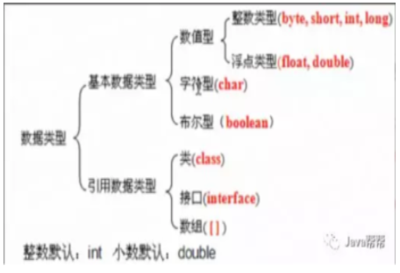Byte    --  一个字节  21*8次方

Short   --  两个字节  22*8次方

Int     --  四个字节  24*8次方

Long   --  八个字节  28*8次方

Char   --  两个字节  22*8次方

Float   --  四个字节  24*8次方

Double  -- 八个字节  28*8次方

2) 定义类：Test1，定义main()方法，分别定义8种数据类型的变量，并赋初始值；然后打印每种类型的变量。

public class Test1 {

//定义主方法

public static void main(String[] args) {

//在控制台上打印

byte a = 1;

short b = 2;

int c = 3;

long d = 4;

char e = '5';

float f = 4.5f;

double g = 5.43423;

boolean h = true;

boolean i = false;

System.out.println(a);

System.out.println(b);

System.out.println(c);

System.out.println(d);

System.out.println(e);

System.out.println(f);

System.out.println(g);

System.out.println(h);

System.out.println(i);

}

}

3) 定义类：Test2，定义main()方法，请按如下要求编写代码：

a) 定义一个byte类型的变量v1，赋初始值；

v1赋给一个int类型的变量v2

v2赋给一个long类型的变量v3

v3赋给一个double类型的变量v4

b) 打印每个变量的值；

public class Test2 {

//定义主方法

public static void main(String[] args) {

//在控制台上打印

byte v1 = 5;

int v2 =v1;

long v3 = v2;

double v4 = v3;

System.out.println(v1);

System.out.println(v2);

System.out.println(v3);

System.out.println(v4);

}

}

4) 定义类：Test3，定义main()方法，请按如下要求编写代码：

a) 定义一个double类型的变量v1，赋值为：3.1

public class Test3 {

//定义主方法

public static void main(String[] args) {

//在控制台上打印

double v1 = 3.1;

double v2 = 88.88;

int v3 =(int)v1;

int v4 = (int)v2;

System.out.println(v3);

System.out.println(v4);

}

}

### 3.1.2 分析以下需求，并用代码实现

1.键盘录入一个三位整数数，请分别获取该三位数上每一位的数值

2.例如：键盘录入的整数123的个位、十位、百位，分别是321

3.打印格式:"数字123的个位是 3, 十位是 2, 百位是 1"

public class HelloWorld {

//定义主方法

@SuppressWarnings("resource")

public static void main(String[] args) {

//在控制台上打印

Scanner sc = new Scanner(System.in);

System.out.println("请输入一个三位数的数值");

int a = sc.nextInt();

int b = a%100%10;

int c = a%100/10;

int d = a/100;

System.out.println("数字a的个位是"+b+", 十位是"+c+", 百位是"+d);

}

}

### 3.1.3 分析以下需求，并用代码实现

1.键盘录入三个int类型的数字

2.要求：

(1)求出三个数中的最小值并打印

(2)求出三个数的和并打印

public class Test {

@SuppressWarnings("resource")

public static void main(String[] args) {

Scanner sc = new Scanner(System.in);

System.out.println("请依次输入三个数值");

int a = sc.nextInt();

int b = sc.nextInt();

int c = sc.nextInt();

//求出三个数中的最小值并打印

int temp = a<b?a:b;

int min = temp<c?temp:c;

System.out.println(min);

//求出三个数的和并打印

System.out.println(a+b+c);

}

}

### 3.1.4 知识点：算数运算符

1) 熟练掌握算数运算符的使用；

1) 定义类：Test4，定义main()方法，请按如下要求编写代码：

a) 定义一个int类型的变量v1，初始值：10

b) 请分别打印这两个数加，减，乘，除，取模的结果

c) v1自增1，将v2自减1

d) 再次打印这两个数：加，减，乘，除，取模的结果

public class Test {

@SuppressWarnings("resource")

public static void main(String[] args) {

int v1 = 10;

int v2 = 3;

//b)打印这两个数加，减，乘，除，取模的结果

System.out.println(v1+v2);

System.out.println(v1-v2);

System.out.println(v1*v2);

System.out.println(v1/v2);

System.out.println(v1%v2);

//将v1自增1，将v2自减1

//System.out.println(v1++);

System.out.println(++v1);

//System.out.println(v2--);

System.out.println(--v2);

//再次打印这两个数：加，减，乘，除，取模的结果

System.out.println(v1+v2);

System.out.println(v1-v2);

System.out.println(v1*v2);

System.out.println(v1/v2);

System.out.println(v1%v2);

}

}

### 3.1.5 知识点：赋值运算符

1) 熟练掌握赋值运算符的使用；

1) 定义类：Test5，定义main()方法，请使用赋值运算符编写代码：

a) 定义一个int类型的变量v1，初始值：10

b) 将此变量加10

c) 将此变量减5

d) 将此变量乘3

e) 将此变量除以10

f) 将此变量模2

g) 打印v1的值

package StudyJavaSE;

/**

* 1)请使用赋值运算符编写代码：

*  a)定义一个int类型的变量v1，初始值：10

*  b)将此变量加10

*  c)将此变量减5

*  d)将此变量乘3

*  e)将此变量除以10

*  f)将此变量模2

*  g)打印v1的值

*

* @author 奋斗蒙

*

*/

public class FuZhiYunsuanfu {

public static void main(String[] args) {

//a)定义一个int类型的变量v1，初始值：10

int a1 = 10;

int a2 = 10;

System.out.println(a1);

System.out.println(a2);

//b)将此变量加10

a1+=10;

System.out.println(a1);

a2 = a2 + 10;

System.out.println(a2);

//c)将此变量减5

a1-=5;

System.out.println(a1);

a2 = a2 - 5;

System.out.println(a2);

//d)将此变量乘3

a1*=3;

System.out.println(a1);

a2 = a2 * 3;

System.out.println(a2);

//e)将此变量除以10

a1/=10;

System.out.println(a1);

a2 = a2 / 10;

System.out.println(a2);

//f)将此变量模2

a1%=2;

System.out.println(a1);

a2 = a2 % 2;

System.out.println(a2);

}

}

### 3.1.6 知识点：比较运算符

1) 熟练掌握比较运算符的使用；

1) 定义类：Test6，定义main()方法，请按如下要求编写代码：

a) 定义一个int类型的变量v1，初始值：10

b) 请打印v1是否等于v2

c) 请打印v1是否大于v2

d) 请打印v1是否小于v2

e) 请打印v1是否大于等于v2

f) 请打印v1是否小于等于v2

g) 请打印v1是否不等于v2

public class Test {

@SuppressWarnings("resource")

public static void main(String[] args) {

//a)定义一个int类型的变量v1，初始值：10

//定义一个int类型的变量v2，初始值：3

int v1 = 10;

int v2 = 3;

//b)请打印v1是否等于v2

System.out.println(v1==v2);

//c)请打印v1是否大于v2

System.out.println(v1>v2);

//d)请打印v1是否小于v2

System.out.println(v1<v2);

//e)请打印v1是否大于等于v2

System.out.println(v1>=v2);

//f)请打印v1是否小于等于v2

System.out.println(v1<=v2);

//g)请打印v1是否不等于v2

System.out.println(v1!=v2);

}

}

### 3.1.7 知识点：逻辑运算符

1) 熟练掌握逻辑运算符的使用；

1) 定义类：Test7，定义main()方法，请按如下要求编写代码：

a) 定义一个int类型的变量v1，初始值：24

b) 请打印：v1 是否大于20，并且小于50

c) 请打印：v1是否小于20，或者大于50

d) 请打印：v1是否大于20，并且v2小于3.5

e) 请使用“非运算符”判断：v1是否不等于25

package StudyJavaSE;

/**

* 请按如下要求编写代码：

* a)定义一个int类型的变量v1，初始值：24

* 定义一个double类型的变量v2，初始值：3.14

* b)请打印：v1 是否大于20，并且小于50

* c)请打印：v1是否小于20，或者大于50

* d)请打印：v1是否大于20，并且v2小于3.5

* e)思考：请使用“非运算符”判断：v1是否不等于25

* @author 奋斗蒙

*

*/

public class LuoJiYunsuanfu {

public static void main(String[] args) {

//a)定义一个int类型的变量v1，初始值：24

//定义一个double类型的变量v2，初始值：3.14

int v1 = 24;

double v2 = 3.14;

//b)请打印：v1 是否大于20，并且小于50

System.out.println((v1>10)&(v1<50));

//c)请打印：v1是否小于20，或者大于50

System.out.println((v1<20)|(v1>50));

//d)请打印：v1是否大于20，并且v2小于3.5

System.out.println((v1>20)&(v2<3.15));

}

}

### 3.1.8 知识点：三元运算符

1) 熟练掌握三元运算符的使用；

1) 定义类：Test8，定义main()方法，请按如下要求编写代码：

a) 定义一个int类型的变量v1，初始值：20

b) 请使用三元运算符求两个数的最大值，并打印；

public class Test {

@SuppressWarnings("resource")

public static void main(String[] args) {

//  a)定义一个int类型的变量v1，初始值：20

//  定义一个int类型的变量v2，初始值：18

int v1 = 20;

int v2 = 18;

//  b)请使用三元运算符求两个数的最大值，并打印；

System.out.println(v1>v2?v1:v2);

}

}

2) 定义类：Test9，定义main()方法，请按如下要求编写代码：

a) 定一个int类型的变量v1，初始值20

b) 请使用三元运算符判断：如果v1大于20v2的值为：大于，

c) 打印v2的值

public class Test {

@SuppressWarnings("resource")

public static void main(String[] args) {

//a)定一个int类型的变量v1，初始值20；

//定义一个String类型的变量v2，初始值：“”

int v1 = 20;

String v2 = "";

//b)请使用三元运算符判断：如果v1大于20，v2的值为：大于，

//否则，将v2的值赋值为：不大于。

//c)打印v2的值

System.out.println(v1>20?(v2="大于"):(v2="不大于"));

}

}

## 3.2 代码题

### 3.2.1 在控制台打印如下的信息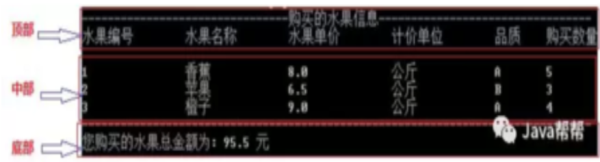l 要求:

l 1.清单顶部为固定的数据，直接打印即可

l 2.清单中部为商品，为变化的数据，需要记录水果信息后，打印

l 3.清单底部包含了统计操作，需经过计算后，打印

/*

-----------------------购买的水果信息--------------------------

1   香蕉  8.0   公斤  A   5

2   苹果  6.5   公斤  B     3

3   橙子  9.0   公斤  A     4

---------------------------------------------------------------

1.定义318个变量用来记录水果信息

2.计算购买的水果总金额

*/

public class Demo01{

public static void main(String[] args){

//1.定义318个变量用来记录水果信息

//香蕉

int bananaId = 1;

String bananaName = "香蕉";

double bananaPrice = 8.0;

String bananaUnit = "公斤";

char bananaLevel = 'A';

int bananaNumber = 5;

//苹果

int appleId = 2;

String appleName = "苹果";

double applePrice = 6.5;

String appleUnit = "公斤";

char appleLevel = 'B';

int appleNumber = 3;

//橙子

int orangeId = 3;

String orangeName = "橙子";

double orangePrice = 9.0;

String orangeUnit = "公斤";

char orangeLevel = 'A';

int orangeNumber = 4;

//2.计算购买的水果总金额

double totalMoney = bananaPrice * bananaNumber + applePrice * appleNumber + orangePrice * orangeNumber;

System.out.println("--------------------------------购买的水果信息----------------------------------");

System.out.println("水果编号 水果名称 水果单价 计价单位 品质 购买数量");

System.out.println(bananaId+"  "+bananaName+"  "+bananaPrice+"  "+bananaUnit+"  "+bananaLevel+" "+bananaNumber);

System.out.println(appleId+"  "+appleName+"  "+applePrice+"  "+appleUnit+"  "+appleLevel+" "+appleNumber);

System.out.println(orangeId+"  "+orangeName+"  "+orangePrice+"  "+orangeUnit+"  "+orangeLevel+" "+orangeNumber);

System.out.println("--------------------------------------------------------------------------------");

System.out.println("您购买的水果总金额为: " + totalMoney +" ");

}

}Java帮帮学习群生态

Java帮帮学习群生态

 Java学习群Java学习群与大牛一起交流 大数据学习群大数据学习群在数据中成长 九点编程学习群九点编程学习群深夜九点学编程 python学习群python学习群人工智能,爬虫
Java帮帮生态承诺

Java帮帮生态承诺